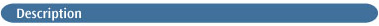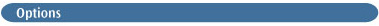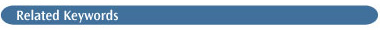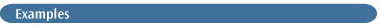# DensityBy default, population and other analysis procedures use the SCF density (i.e., the Hartree-Fock density for post-SCF methods; the DFT density for DFT jobs, and the CASSCF density for CAS jobs). The generalized densities for the MP2, MP3, MP4(SDQ), QCISD, CCD, CCSD, CID and CISD and SAC-CI methods are available. These are based on the Z-Vector [140,445,446,447], and hence yield multipole moments which are the correct analytical derivatives of the energy. The unrelaxed densities at second order (not the same as MP2) can also be used but are not recommended.

The options of the Density keyword select which density to analyze. The Density keyword without an option is equivalent to Density=Current.Current
Use the density matrix for the current method. This is the default when no option is given to Density.

All
Use all available densities. This is allowed for population analysis but not for electrostatics or density evaluation. Note that this option does not produce densities for all of the excited states in a CI-Singles calculation, only the density for the state of interest (see the examples below for a method of doing the former).

SCF
Use the SCF density. HF is a synonym for SCF.

MP2
Use the generalized density corresponding to the second-order energy.

Transition=N or (N,M)
Use the CIS transition density between state M and state N. M defaults to 0, which corresponds to the ground state.

AllTransition
Use all available CIS transition densities.

CI
Use the generalized density corresponding to the CI energy.

QCI
Use the generalized density corresponding to the QCI (or coupled cluster) energy. CC is a synonym for QCI.

RhoCI
Use the one-particle density computed using the CI wavefunction for state N. This is not the same as the CI density , and its use is discouraged! Chapter 9 of Exploring Chemistry with Electronic Structure Methods discusses this issue .

Rho2
Use the density correct to second-order in Møller-Plesset theory. This is not the same as the MP2 density, and its use is discouraged! 

CIS=N
Use the total unrelaxed CIS density for state N. Note that this is not the same as the density resulting from CIS(Root=N,...) Density=Current, which is to be preferred .

Checkpoint
Recover the density from the checkpoint file for analysis. Implies Guess=Only ChkBasis: the calculation does not recompute new integrals, SCF, and so on, and retrieves the basis set from the checkpoint file.The following route section specifies a CI-Singles calculation which predicts the first six excited states of the molecule under investigation. The population and other analyses will use the CIS density corresponding to the lowest excited state:

```%Chk=benzene
# CIS(NStates=6)/6-31+G(d,p) Density=Current Pop=CHelpG ```

The following route section may be used to rerun the post-CIS analyses for the other excited states:

```%Chk=benzene
# CIS(Read,Root=N) Density=Current Pop=CHelpG
# Guess=Read Geom=AllCheck ```

This route picks up the converged CIS and CIS wavefunction from the checkpoint file, and performs the necessary CPHF calculation to produce the relaxed density for state N, which is then used in the population and other analyses.